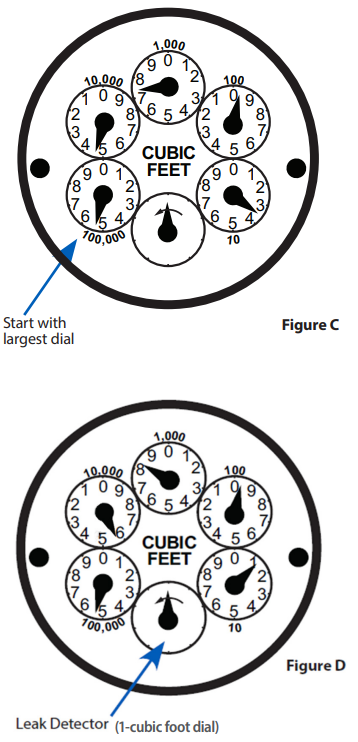All EBMUD customers have a meter that measures their water use. Water bills reflect how much water was used since the last meter reading. Meters measure cubic feet of water used (one cubic foot equals 7-1/2 gallons). EBMUD bills in units of 100 cubic feet (748 gallons). Meters are usually in the ground under a cement cover (with an EBMUD logo) by the street near the curb.

If you get a high bill, a meter test will tell you if you have a leak, and can help you pinpoint the location (indoors or out).

By tracking your water use, you’ll know what activities or fixtures are using water, when, and how much, so you can make informed choices about how you use water. For example, if you have sprinklers, you can read your meter before and after an irrigation cycle to find out how much water is used each time you water your garden.

If you read your meter often, you are more likely to catch spikes in water use quickly, making it more likely you’ll catch a leak early.

### What is a water meter?

Water meters are essentially odometers recording how much water is used at your home or business. Reading your meter between bills is a great way to track water use and catch leaks. Water meters are usually located in the front of your property in a cement or metal box. Use a channel lock wrench or a big screwdriver to pry open the lid. Inside the box and underneath a black plastic cover is the meter which has moving numbers. These numbers will increase as water flows from the street to your property. Meters record water use in cubic feet. One cubic foot = 7.48 gallons. EBMUD bills in units of 100 cubic feet, dropping down the last two digits. One unit = 748 gallons.

#### Straight Meters

Step 1:Open your meter box and write down all the meter numbers on the dial. This is called the meter read. Return the following day, week, or month, and record a second read.

• In Figure A, the read is 547030 (5470 billing units).

• In Figure B, the read is 548010 (5480 billing units).

Step 2: Subtract the first reading from the second reading. Ex: 548010 - 547030 = 980 cubic feet.

Step 3: Convert to gallons by multiplying by 7.48. Ex: 980 cubic feet x 7.48 = 7,330 gallons.

Step 4: Determine how many gallons per day you used between readings. Divide the total number of gallons by the number of days between the two readings. In this example, 21 days passed between readings. Ex: 7,330 / 21 days = 349 gallons per day (gpd). Step 5: Calculate average use per person per day. Divide gpd by number of people in the household. Ex: 349 gpd divided by 4 people in the house = 87 gpd/ person. Efficient per day per person water use should be between 35 to 50 gpd per person for indoor use.

#### Round-Dial Meters

Some EBMUD meters may have multiple dials. These meters are known as “Round Dial Meters” or “Round-Reading Meters.” The image to the left (Figure C) has 6 dials. The 100-, 10-, and 1- cubic foot dials are not used for billing but are helpful for leak detection. The hands on individual dials may rotate either clockwise or counter clockwise.To read the meter, start with the dial labeled 100,000 and read clockwise to the next dial labeled 10,000, then the 1,000 dial, the 100 dial, and finally the 10 dial. If the hand on any dial is between two numbers, use the lower number.

• In Figure C, the read is 54793 (547 billing units).

• In Figure D, the read is 55801 (558 billing units).

Step 1: Subtract the first reading from the second reading. Ex: 55,801 - 54,793 = 1,008 cubic feet.

Step 2: Convert to gallons by multiplying by 7.48. Ex: 1,008 cubic feet x 7.48 = 7,540 gallons.

Step 3: Determine how many gallons per day you used between readings. Divide the total number of gallons by the number of days between the two readings. In this example, 21 days passed between readings. Ex: 7,540 / 21 days = 359 gallons per day (gpd).

Step 4: Calculate average use per person per day. Divide gpd by number of people in the household. Ex: 359 gpd divided by 4 people in the house = 90 gpd/person. Efficient per day per person water use should be between 35 to 50 gpd per person for indoor use.

### How to detect leaks

The 10-cubic foot and 1-cubic foot dials can be used to detect leaks. If there is no water use, the 10-cubic foot and 1-cubic foot dials should not move. If these dials move and there is no water use, that would indicate a possible leak

### Water Leaks and High Bills

If you have a higher than average water bill, you may have a leak. Leaks can occur in toilets, pipes, faucets, water heaters, ice machines, dishwashers, washing machines, and irrigation systems.